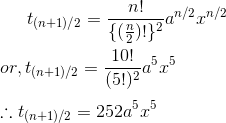# Binomial Theorem and Expansion of Binomial Expression

The expression consisting of two terms is known as binomial expression. For example,

```a+b
x+y
```

Binomial expression may be raised to certain powers. For example,

```(x+y)2
(a+b)5
```

## Expansion of Binomial Expression

In order to expand binomial expression, we use repeated multiplication. For example,

```(a+b)2
= (a+b)(a+b)
= a(a+b) + b(a+b)
= a2 + 2ab + b2

(m+n)3
= (m+n)(m+n)2
= (m+n)(m2 + 2mn + n2)
= m(m2 + 2mn + n2) + n(m2 + 2mn + n2)
= m3 + 3m2n + 3mn2 + n3
```

## Binomial Theorem

When power of expression increases, complexity of calculation of binomial expansion increases.To solve this problem, Isaac Newton introduced a theorem known as binomial Theorem.

### Binomial Theorem Statement

For any positive integer n,

`(a+x)n = C(n,0)an + C(n,1)an-1x + C(n,2)an-2x2 + ... + C(n,r)an-1xr+ ... + C(n,n)xn `

### Proof of Binomial Theorem

Binomial theorem can be proved by using Mathematical Induction.

Principle of Mathematical Induction

Mathematical induction states that, if P(n) be a statement and if

1. P(n) is true for n=1,
2. P(n) is true for n=k+1 whenever P(n) is true for n=k.

then P(n) is true for all natural numbers n.

Now, let P(n) be the given statement. Then,

```When n=1,
LHS = a+x
RHS = C(1,0)a+C(1,1)a1-1x = a+x
Hence, P(1) is true.```

Let us assume that P(n) is true for some value n=k. Then,

`(a+x)k = C(k,0)ak + C(k,1)ak-1x + C(k,2)ak-2x2 + ... + C(k,r)ak-1xr+ ... + C(k,k)xk `

Multiplying both sides by (a+x), we get

```  (a+x)k+1
= (a+x)[C(k,0)ak + C(k,1)ak-1x + C(k,2)ak-2x2 + ... + C(k,r)ak-1xr+ ... + C(k,k)xk]
= C(k,0)ak+1 + C(k,1)akx + C(k,2)ak-1x2 + ... + C(k,k)axk + C(k,0)akx + C(k,1)ak-1x2 + ... + C(k,k)xk+1
= ak+1 + [c(k,1) + c(k,0)]akx + [c(k,2) + c(k,1)]ak-1x2 + [c(k,3) + c(k,2)]ak-2x3 + ... + xk+1
= C(k+1, 0)ak+1 + C(k+1, 1)akx + C(k+1, 2)ak-1x2 + ... + C(k+1, r)ak+1-rxr + C(k+1, k+1)xk
```

Hence, P(k+1) is true whenever P(k) is true.

So, by principle of mathematical induction P(n) is true for all natural numbers n, i.e.

`(a+x)n = C(n,0)an + C(n,1)an-1x + C(n,2)an-2x2 + ... + C(n,r)an-1xr+ ... + C(n,n)xn `

Similarly,

```(a-x)n = C(n,0)an - C(n,1)an-1x + C(n,2)an-2x2 - . . . + (-1)rC(n,r)an-1xr+ ..... + (-1)nC(n,n)xn
```

### General Term in Binomial Expression

The general term in the expansion of (a+x)n is (r+1)th term i.e.

```tr+1 = C(n,r)an-rxr

Thus, First term(r=0), t1 = C(n,0)an
Second term(r=1), t2 = C(n,1)an-1x1 and so on.```

Now, the binomial theorem may be represented using general term as,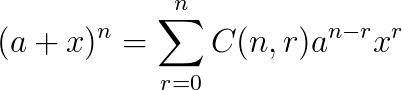Middle term of Expansion

In order to find the middle term of the expansion of (a+x)n, we have to consider 2 cases.

1. When n is even: When n is even, suppose n = 2m where m = 1, 2, 3, …

Then, number of terms after expansion is 2m+1 which is odd. Thus, it has only one middle term which is (m+1)th term. So,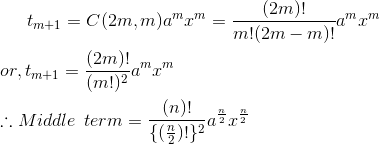2. When n is odd: When n is odd, suppose n = 2m-1 where m = 1, 2, 3, …

Then, number of terms after expansion is 2m which is even. Thus, it has 2 middle terms which are mth and (m+1)th terms. So,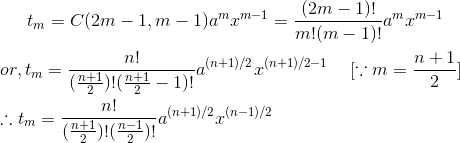## Binomial Expansion Examples

1. Expand (a+b)5 using binomial theorem.

Solution:

Here, the binomial expression is (a+b) and n=5.

So, using binomial theorem we have,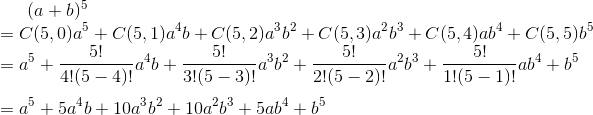2. Find the middle term of the expansion (a+x)10.

Solution:

Since, n=10(even) so the expansion has n+1 = 11 terms. Hence there is only one middle term which is